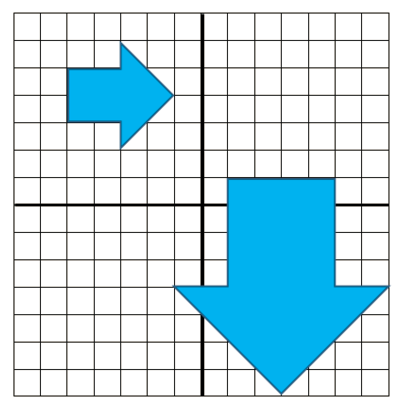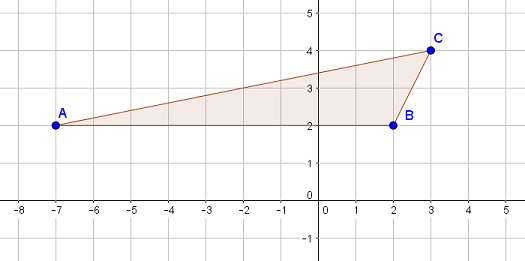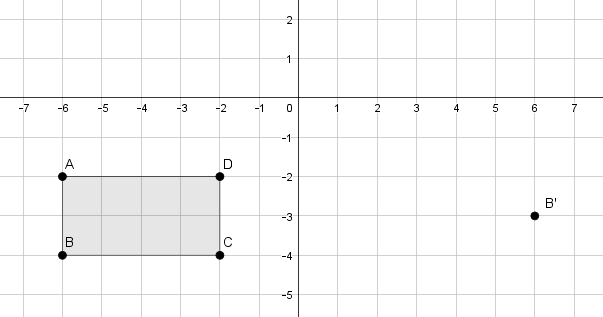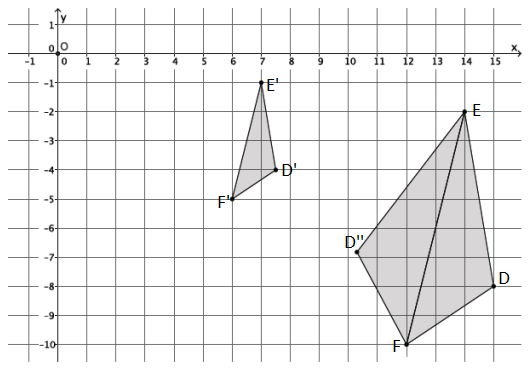# Transformations and Angle Relationships

## Objective

Describe a sequence of dilations and rigid motions between two figures. Use coordinate points to represent relationships between similar figures.

## Common Core Standards

### Core Standards

?

• 8.G.A.3 — Describe the effect of dilations, translations, rotations, and reflections on two-dimensional figures using coordinates.

• 8.G.A.4 — Understand that a two-dimensional figure is similar to another if the second can be obtained from the first by a sequence of rotations, reflections, translations, and dilations; given two similar two-dimensional figures, describe a sequence that exhibits the similarity between them.

?

• 7.G.A.1

• 7.RP.A.2

## Criteria for Success

?

1. Determine if two figures are similar by describing a sequence of dilations and other rigid motions that maps one figure to another.
2. Describe sequences of transformations between similar figures; find more than one sequence of transformations.
3. Determine new coordinates of points that undergo dilations and other transformations.

## Tips for Teachers

?

In this lesson, students combine their understanding of dilations and rigid transformations to determine if two figures are similar in the coordinate plane.

#### Fishtank Plus

• Problem Set
• Student Handout Editor
• Vocabulary Package

## Anchor Problems

?

### Problem 1

Determine using rotations, translations, reflections, and/or dilations whether the two polygons shown below are similar.#### References

Illustrative Mathematics Are They Similar?

Are They Similar?, accessed on Oct. 13, 2017, 4:11 p.m., is licensed by Illustrative Mathematics under either the CC BY 4.0 or CC BY-NC-SA 4.0. For further information, contact Illustrative Mathematics.

Modified by The Match Foundation, Inc.

### Problem 2

Triangle ${ABC}$, shown on the coordinate plane below, is dilated from point $A$ by a scale factor of ${\frac{1}{2}}$ and then translated $3$ units down and $2$ units left.

What are the new coordinates for point ${B'}$?#### References

EngageNY Mathematics Grade 8 Mathematics > Module 3 > Topic A > Lesson 6Teacher Version: Exit Ticket

Grade 8 Mathematics > Module 3 > Topic A > Lesson 6 of the New York State Common Core Mathematics Curriculum from EngageNY and Great Minds. © 2015 Great Minds. Licensed by EngageNY of the New York State Education Department under the CC BY-NC-SA 3.0 US license. Accessed Dec. 2, 2016, 5:15 p.m..

### Problem 3

Rectangle ${{ABCD}}$ and point ${B'}$ are shown in the coordinate plane below. Rectangle ${{ABCD}}$ underwent two transformations, one of which was a dilation.Describe a possible sequence of transformations that could result in point $B$ mapping to point ${B'}$.

## Problem Set

?The following resources include problems and activities aligned to the objective of the lesson that can be used to create your own problem set.

?

In the picture below, triangle ${{DEF}}$ has been dilated from the origin by a scale factor of ${\frac{1}{2}}$ and is named ${{D'E'F}'}$. There is also triangle ${{D''EF}}$, which is congruent to triangle ${{DEF}}$. Describe a sequence that would map triangle ${D'E'F}$ onto triangle ${{D''EF}}$.#### References

EngageNY Mathematics Grade 8 Mathematics > Module 3 > Topic B > Lesson 8Exit Ticket

Grade 8 Mathematics > Module 3 > Topic B > Lesson 8 of the New York State Common Core Mathematics Curriculum from EngageNY and Great Minds. © 2015 Great Minds. Licensed by EngageNY of the New York State Education Department under the CC BY-NC-SA 3.0 US license. Accessed Dec. 2, 2016, 5:15 p.m..

Modified by The Match Foundation, Inc.

?